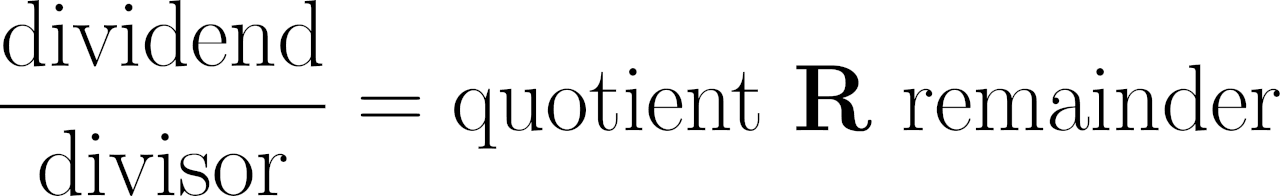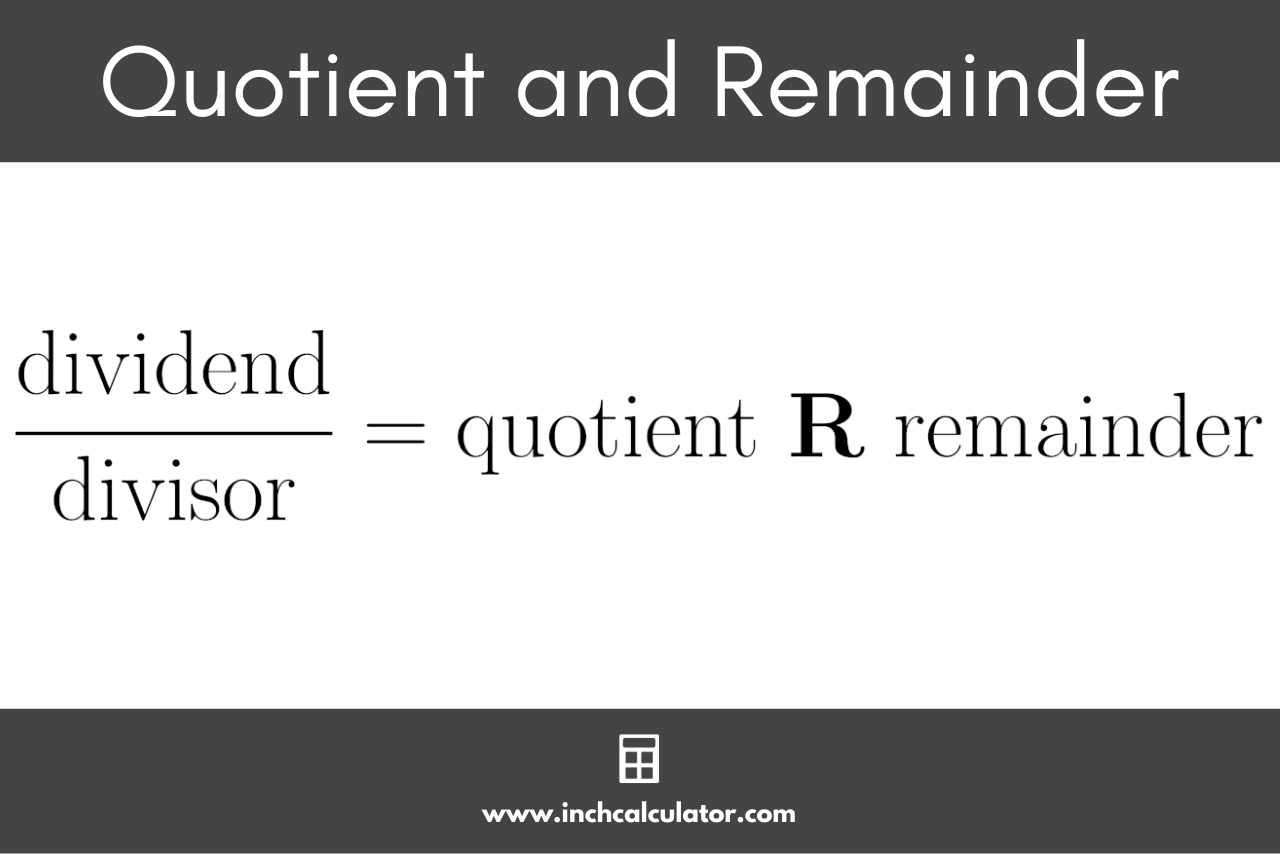# Remainder Calculator

Calculate the quotient and remainder of a division problem by entering the dividend and divisor in the remainder calculator below.## Result:

 Quotient: 6 Remainder: 3 Full Answer: 6 R 3

### Solution

45 ÷ 7 = 6.429

#### Step Two: Find the Quotient Integer

integer value of 6.429 is 6

#### Step Three: Find the Remainder

remainder = dividend - (divisor × quotient integer)

remainder = 45 - (7 × 6) = 3

#### Step Four: Put the Full Answer Together

full answer = 6 R 3

Learn how we calculated this below

## How to Find the Remainder

Whether you’re performing a standard division operation or performing long division, there are a few parts to the division problem, as shown in the graphic above.

The dividend is the number being divided and is the first number in a division problem, either above the division line or to the left of the division symbol (÷).

The divisor is the number being divided by and is the second number in a division problem, either below the division line or to the right of the division symbol.

The quotient of the solution is the whole number part of the solution.

The remainder is the remaining part of the solution, or what’s left over.You can calculate the quotient and remainder of the division problem in a few steps.

### Step One: Calculate the Result as a Decimal

The first step is to calculate the result as a decimal value. Using a calculator, divide the dividend by the divisor.

For example, let’s find the quotient and remainder of 45 divided by 7.

45 / 7 = 6.429

### Step Two: Find the Quotient as an Integer

The next step is to separate the result into a whole number, or integer, and the remaining decimal value. The whole number will be the result’s quotient.

Continuing the example above, let’s find the quotient from the result of the division problem above (6.429).

6.429 as an integer = 6

### Step Three: Calculate the Remainder

You can calculate the remainder by multiplying the quotient by the divisor, then subtracting that from the dividend.

Continuing the example, let’s find the remainder of 45 divided by 7, given the quotient of 6.

remainder = 45 – (7 × 6) = 3

### Step Four: Complete the Solution

Now, you can put the answer together in the form quotient R remainder.

To complete the example, let’s find the full solution of 45 divided by 7 using the quotient and remainder we found above.

full answer = 6 R 3

### What is a remainder in math?

The remainder of a division problem is the value left over when one number is not evenly divisible into another. It is the partial value in the result that is after the decimal point.

### What is the quotient remainder formula?

The division formula to calculate the quotient and remainder is:

dividend ÷ divisor = quotient and remainder

### Can the remainder contain a decimal?

When performing division problems using a quotient and remainder, you will not use any decimal numbers; thus, the resulting remainder should not contain a decimal.

It is possible to express the leftover value that cannot be divided evenly as a fraction or decimal, but this would not typically be called the remainder.